Thank you for visiting nature.com. You are using a browser version with limited support for CSS. To obtain the best experience, we recommend you use a more up to date browser (or turn off compatibility mode in Internet Explorer). In the meantime, to ensure continued support, we are displaying the site without styles and JavaScript.

# The evolution of the G matrix: selection or drift?

## Abstract

The evolution of quantitative characters can be described by the equation Δ=GP−1S where Δ is the vector of mean responses, G is the matrix of additive genetic variances and covariances, P is the matrix of phenotypic variances and covariances and S is the vector of selection differentials. This equation can be used to predict changes in trait values or to retrospectively estimate the selection gradient and is thus a central equation of evolutionary quantitative genetics. Genetic variances and covariances will be reduced by stabilizing selection, directional selection and drift, and increased by mutation. Changes in trait values resulting from directional selection that are comparable with differences observed among species are readily obtainable in short geological time spans (<5000 generations) with selection intensities so small that they would have an insignificant effect on the G matrix (of course it is possible that such changes came about by strong selection over a few generations, followed by long periods of stasis; there is insufficient evidence to presently distinguish these two possibilities). On the other hand, observed effective population sizes are sufficiently small that considerable changes in G can be expected from drift alone. The action of drift can be distinguished from selection because the former produces a proportional change in G whereas the latter, in general, will not. A survey of studies examining variation in G suggests that the null hypothesis that most of the variation can be attributed to drift rather than selection cannot be rejected. However, more research on the predicted statistical distribution of G as a result of selection and/or drift is required and further development of statistical tests to distinguish these two forces needs to be made.

## What is the G matrix?

Quantitative genetics has long made significant contributions to the development of domesticated plant and animal species. In the last two decades there has been an increasing interest in the use of quantitative genetics to understand the evolution of quantitative traits in natural populations. For domestic organisms the central equation of quantitative genetics is the relationship, R = h2S (response = heritability × selection differential), or less frequently the two-trait formulation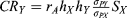, where CRY is the correlated response of trait Y to direct selection on trait X, rA is the genetic correlation between X and Y, and σPi is the phenotypic standard deviation of trait i. However, natural selection does not typically act upon single or even pairs of traits, but rather the whole organism and hence it is more appropriate to consider the multivariate extension of the above equations (Lande, 1979a), Δ=GP−1S where Δ is the vector of mean responses (conventionally symbolized by z), G is the matrix of genetic variances and covariances, P is the matrix of phenotypic variances and covariances, and S is the vector of selection differentials. The matrix combination GP–1 can thus be viewed as the multivariate ‘equivalent’ of h2, with G replacing the additive genetic variance in the numerator and P–1 replacing the phenotypic variance in the denominator.

## Of what use is G?

The multivariate response equation has been used theoretically to explore evolutionary trajectories with particular reference to constraints on the direction of evolutionary change (e.g. Via & Lande, 1985; Arnold, 1992; Bjorklund, 1996; Schluter, 1996). With respect to the analysis of natural populations, the response equation has been used to retrospectively reconstruct the selection regime that has given rise to a change over time or among populations (Arnold, 1992), or to predict responses to selection (Morris, 1971; Grant & Grant, 1993; Roff & Fairbairn, 1999). Retrospective selection analysis assumes that both G and P remain constant and that there are no dramatic reversals in the direction of selection (Shaw et al., 1995). Depending upon the nature of the analysis, prediction of response requires either that both G and P remain constant or change in a proportional manner relative to the original matrices.

There are two defences for the assumption of constancy. The first is the same as applied to artificial selection experiments, namely that although selection will change allelic frequencies and hence genetic variance and covariances (and thus also their phenotypic counterparts), the change will be so small per generation that the equations will remain correct at least for a dozen or so generations (see Roff, 1997 for a review providing support for this assumption). This argument clearly cannot be applied to those cases in which the equations are used to predict changes taking place over hundreds or thousands of generations. For this the assumption is made that directional or stabilizing selection is sufficiently weak that any erosion of variance is countered by new variation added by mutation (Lande, 1976a).

## How weak is weak selection?

We can examine this question by asking what selection is necessary to produce a change in mean trait value that is characteristic of some given taxonomic level. A survey of tests of variation in G matrices at different taxonomic levels suggests that statistically significant differences are found at least at the level of species (Roff & Mousseau, 1999). Most studies have focused upon morphological traits and thus we must ask how much variation is characteristically found among species within any given genus. An analysis of variation among species within 32 different genera covering a wide range of animals suggests that a typical range is a two- to four-fold difference in linear dimensions, though differences larger than tenfold also occur (Fig. 1). For illustration, suppose that h2 = 0.5 (a typical heritability for morphological traits), VA = 25 and the initial trait value is 50. When the top 99.9% of the population is selected each generation then after 5000 generations the trait value will be 50 + (5000)(0.00337) (√[0.5]) = 110, a two-fold increase, while if the top 99% is selected the trait value will be 50 + (5000)(0.02692)(√[0.5]) = 526, a 10-fold increase. Both of these changes are within the range of interspecific variation in morphological traits and the former is probably most typical (see above). On a geological time-scale 5000 generations is short and changes of the above magnitude would be considered rapid. A cull of 0.1% of the population per generation is extremely weak (certainly below present levels of detection for most studies). Lande (1976b) estimated the required cull rate per generation to account for change in the tooth dimensions during the evolution of the horse: to obtain the observed changes requires selective deaths of the order of 2 per million individuals, which is considerably weaker than the above rates (99.9998% survive), but the time-span is in the millions rather than thousands of generations. Of course, it is unlikely that selection acts at a constant, minute rate each generation but the important point is that even very low rates of selection can produce very large phenotypic changes in what is no more than a geological ‘instant’. Thus the hypothesis of weak selection is at least tenable, but requires empirical support (Turelli, 1988).

Endler (1986; p. 210) surveyed a large number of studies in which estimates of selection intensities in natural populations were made and found that ‘natural selection is often as strong as artificial selection’. This result does not, however, demonstrate that the assumption of weak selection is invalid. First, it remains to be shown if these are typical or simply those that have been selected a priori because the researchers suspected that the traits in question would be under strong selection. There is little value in selecting traits for which selection intensities cannot be measured although these may represent the majority of traits. Secondly, single or short-term episodes of strong selection will not greatly affect the additive genetic variance (Roff, 1997). What we need to know is the frequency with which episodes of strong selection occur.

## How does selection and/or drift alter G?

When genetic correlations arise principally from pleiotropy, populations are large and mating is random, the G matrix is determined by the joint effects of pleiotropic mutation and multivariate selection (Lande, 1980). Changes in the selective regime will produce changes in G but there is no a priori general prediction that can be made (or has so far been published). If selection is strong enough to significantly alter the gene frequencies of the traits subject to selection there will be a change in that portion of the G matrix in which the genes have influence, i.e. the additive genetic variance of the target trait plus the additive genetic covariances between the target trait and other nontarget traits. Thus, in general, the change in the G matrix will show no overall general pattern, but will depend upon the selection regime and the traits included in the matrix. In contrast, genetic drift will cause G matrices to diverge but remain proportional to each other (Lande, 1979a).

Population bottlenecks can convert nonadditive genetic (co)variance into additive genetic (co)variance (Roff, 1997; chapter 8) but the general effect of genetic drift is to erode additive genetic variance. This should be particularly true for morphological traits in which non additive effects are typically small (Mousseau & Roff, 1987; Crnokrak & Roff, 1995; Derose & Roff, 1999). Non-additive effects are present in life history traits (Crnokrak & Roff, 1995; DeRose & Roff, 1999) and hence changes in the G matrix due to short episodes of genetic drift could be unpredictable.

To illustrate the potential importance of genetic drift when there is only additive genetic variance, consider the change in the additive genetic variance of a single trait as a result of drift alone. The proportional decline in the additive genetic variance after t generations is approximately $e - t / 2 N e$ (Robertson, 1960). After 5000 generations the proportional decline for an effective population size of 5000 will be 0.61, which is a substantial reduction in a very short geological period. Thus even very large populations can be subject to significant erosion in additive genetic variance due to genetic drift. A review of estimated effective population sizes produced less than 20% greater than 1000 and over 70% in the range 10–500 (see table 8.3 and figs 8.2 in Roff, 1997). The populations studied do not represent a random sample (there are few invertebrates) but do suggest that random genetic drift could play a very important role in evolutionary changes in many populations (see also Lande, 1979b).

The erosion of additive genetic (co)variance resulting from the combined effects of selection (directional or stabilizing) and drift will be compensated in part or whole by new variation added by mutation. For the infinitesimal model, truncation selection, drift and mutation will change the additive variance, σ2A, after one generation to (Keightley & Hill, 1987)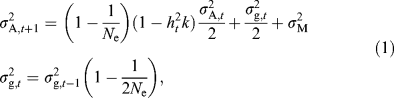where h2t is the heritability at time t, which changes because σ2A changes, k = i(ix), where i is the intensity of selection and x is the standardized deviation of the truncation point of selection and σ2g,t is the genic variance in the population, which is the genetic variance that would be present under linkage and Hardy–Weinberg equilibria. Assuming this to be true in the initial, unselected population we have σ2A,02g,0. The genetic variance introduced by mutation is σ2M. At equilibrium the additive genetic variance maintained by selection, drift and mutation, σ2A, is obtained from the solution to the quadratic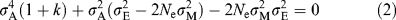which is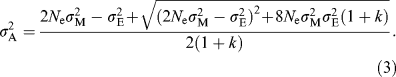From the above it is evident that the equilibrium additive genetic variance is approximately proportional to the effective population size. For example, suppose the proportion culled per generation is 0.1%, and the mutational variance is 0.002, which is within the observed range (see tables 9.7 in Roff, 1997), then for effective population sizes of 10, 100, 1000 the equilibrium additive genetic variance is 0.04, 0.40 and 4.0, respectively. Even if we make selection very strong by culling 50% of the population per generation, the respective additive genetic variances are still approximately proportional to Ne (0.04, 0.34, 2.73, respectively). Bürger (1993), assuming weak selection, obtained the simple result that at equilibrium under directional selection the additive genetic variance would be equal to 2Neσ2M, which is the equilibrium variance under the neutral mutation–drift balance as derived by Lynch & Hill (1986).

## What statistical methods are presently available to test for variation among G matrices?

Given the G matrix of two or more populations consisting of either the same or different species we are in the first instance interested in two questions: first, ‘can we reject the hypothesis that the matrices came from the same statistical population?’. This null hypothesis supposes that the populations do not differ in population size, selection history or mutational variances. We might be inclined to entertain such a hypothesis when comparing several populations of the same species but it seems an unlikely scenario when considering different species. The second question is, ‘can we reject the hypothesis that differences among the G matrices can be accounted for on the basis of drift alone?’ This hypothesis does not preclude the possibility that there are differences in selection history or mutational variances among the populations, but postulates that genetic drift will be relatively so large that it overwhelms such effects. It is important to remember that proportionality of the G matrices does not imply that the mean phenotypes have not been drastically altered by selection. If equality and proportionality can be rejected we accept the hypothesis that differences are, at least in part, a consequence of variation in selection history and/or mutational effects.

The statistical methods proposed to address the above hypotheses can be classified into four groups: element-by-element comparisons, correlation between matrices, maximum likelihood, and the Flury hierarchical comparison. Most analyses have involved only two populations and for simplicity I shall assume that only two matrices are being compared and that the elements of the matrices are ‘lined up’ in two vectors. Element-by-element comparison entails doing a separate test on each element of the matrices (Brodie, 1993; Paulsen, 1996; Roff & Mousseau, 1999; Roff et al., 1999). The simplest such test is to check whether the difference between the elements is greater than expected by chance, which may be done using a bootstrap approach (Paulsen, 1996), randomization (Roff et al., 1999), or a t-test where the standard errors are derived by using the jackknife (Brodie, 1993; Roff, 1997). The problem with the method is that the elements within a matrix are not independent of each other and there are likely to be so many tests that after correction for multiple tests there is too little statistical power to be useful. However, while the method may not serve as a means of testing for overall differences it is useful as a ‘data-exploration’ technique, serving to indicate where large differences might lie.

The most commonly used test is to compare the correlation between the matrices. To circumvent the problem of nonindependence, Lofsvold (1986) suggested the randomization test known as Mantel’s test. This test has been quite widely used although its statistical validity has been questioned (Shaw, 1992). My objection to the method, apart from those enunciated by Shaw (1992) is that it cannot distinguish the hypotheses presented above. A highly significant correlation between matrices could indicate equality (i.e. yi = xi, where yi and xi are the corresponding elements in the two ‘vectorized’ matrices), proportionality (i.e. yi = bxi, where b ≠ 1), or the joint effects of drift and selection/mutation (i.e. yi = a + bxi, where a ≠ 0). The three hypotheses can be distinguished by a regression approach using the reduced major axis method and randomization to estimate the required probabilities (Roff et al., 1999).

Maximum likelihood methods have become particularly popular in quantitative genetic analyses in general; they have been used to address the question of similarity between matrices (e.g. Shaw, 1991; Holloway et al., 1993), but have not been used to distinguish equality from proportionality vs. other types of differences. A method that shows considerable promise and is itself based on maximum likelihood is the Flury approach which considers a hierarchy of possible differences. This method looks at the matrices from the perspective of their common principal components: starting from the ‘top’ of the hierarchy the matrices can be (a) identical, (b) proportional, in which case the matrices share identical principal components but their eigenvalues differ by a proportional constant, (c) they can have one or more, but not all, principal components in common, or (d) they can have completely unrelated structures (Phillips & Arnold, 1999; Fig. 2). The argument for analysing the principle components is that evolutionary change will be influenced by the size of the eigenvalues corresponding to the principal components (Bjorklund, 1996; Schluter, 1996). Eigenvalues that are zero indicate directions in which there is no genetic variation and hence in which there cannot be evolutionary change. Very large eigenvalues indicate highly correlated traits, and thus selection will be strongly influenced by the associated principal component.

Determining that two matrices differ does not itself tell us which process (drift vs. selection) is the more significant. For this purpose we require some method of partitioning the effect that can be attributed to drift vs. that which can be attributed to selection. The following approach based on mean-square deviations is one possible method. First, we compute the mean-square error for the three models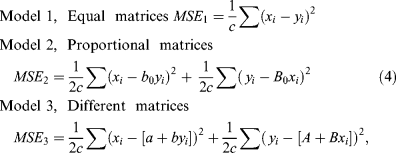where c is the number of variances and covariances, b0 and B0 (= 1/b0) are the slopes of the reduced major axis regression forced through the origin, and a, b, A and B are the parameters of the reduced major axis regression with the intercept included. The MSE is calculated using both ‘x on y’ and ‘y on x’, because these do not each give the same value. We can now ask how much the MSE is reduced by the assumption of a model that only assumes drift (model 2) vs. one that assumes both drift and selection (model 3). To illustrate this I compare the G matrices for two populations of garter snake studied by Arnold & Phillips (1999). Using the Flury hierarchical method the G matrices for females from a coastal population were found to have common principal components but not to be proportional (Arnold & Phillips, 1999). However, it is visually evident that the elements of the matrices do not differ much from proportionality (Fig. 3). This is confirmed by the comparison of the percentage reduction: model 2 accounts for a 17.1% reduction in the mean-square error whereas model 3 accounts for a 18.3% reduction. Thus 17.1% reduction can be attributed to drift while only 1.3% can be attributed to selection. This does not mean that drift actually caused the divergence, for it is possible that selection caused a proportional change in the matrices. However, in general, selection will cause nonproportional changes. Thus, in the absence of evidence to the contrary, the hypothesis that the major force causing divergence was drift cannot be rejected.

## What have the analyses of G matrices told us to date?

Almost all studies have compared either different populations of the same species or different species within the same genus. Most have not distinguished between the effects of drift vs. the effects of selection. More importantly many used a correlational test, which as discussed above, cannot distinguish between equality, proportionality and a general linear relationship. The majority of studies comparing populations have found either no significant differences or significant correlations, whereas comparisons between species have most frequently found differences (Fig. 4). Of course this does not mean that there are no differences between populations, simply that the difference is smaller than can typically be detected given the sample sizes typically used.

Another way of approaching the problem is to ask how much of the difference at the various taxonomic levels is attributable to drift vs. selection. Where significant variation between groups has been found it can almost all be accounted for by drift (Fig. 5). Interestingly, the points showing the greatest ‘effect’ of drift and selection (as indicated by the deviation from the line of equality) are all nonsignificant indicating that analyses should consider both the results of hypothesis testing and interval estimation. Also included in Fig. 5 are the results from a selection experiment on body size in D. melanogaster. Comparison of the G matrices of the base and control populations showed significant differences (Shaw et al., 1995), which, as shown in the plot, can be attributed to drift, as would be expected. Comparisons of the control population with those selected for large or small size suggests that drift has caused even greater deviation of the selected lines from the control. Further, there appears to have been no effect of selection on G in the population selected for small size but an effect in the population selected for large size (Fig. 5). However, these conclusions are suspect because in neither case did the G matrix of the control population differ from that of the selected population (Shaw et al., 1995).

## Where do we go from here?

There is considerable scope for further theoretical analysis of the evolution of the G matrix. In particular, what is the relationship between selection and the deviation from proportionality expected under drift alone? There is also need for more research into both the statistical properties of the proposed tests and further development of methods for estimating the difference between matrices. I have presented one measure, the reduction in mean-squared deviation, which suggests that most of the differentiation observed between populations and species is due to drift rather than selection. Drift is a consequence of differences in effective population size, which are likely to be a consequence of ecological factors: thus we might expect that variation in G should correlate with both taxonomic and ecological variables. Support for the latter is provided by the study of the genetic correlations between morphological characters in the amphipod Gammarus minus (Jernigan et al., 1994).

The G matrix is central to the quantitative genetics of evolutionary processes. Recent developments have shown great promise in improving our ability to analyse variation among matrices. What is presently lacking is an examination of a group incorporating several taxonomic levels (e.g. species and genus) and ecological variables. While such a research programme would be very labour-intensive, the rewards certainly merit the required expenditure.

## References

1. Arnold, S. J. (1992). Constraints on phenotypic evolution. Am Nat, 140S: 85–107.

2. Arnold, S. J. and Phillips, P. C. (1999). Hierarchical comparison of genetics variance-covariance matrices. II. Coastal-inland divergence in the garter snake Thamnophis elegans. Evolution, 53: 1516–1527.

3. Barbour, R. W. and Davis, W. H. (1969). Bats of America. University Press of Kentucky, Lexington, KY.

4. Barker, J., Grigg, G. C. and Tyler, M. J. (1995). A Field Guide to Australian Frogs. Surrey Beatty and Sons, Chipping Norton, NSW, Australia.

5. Beirne, B. P. (1956). Leafhoppers (Homoptera: Cicadellidae) of Canada and Alaska. Can Entomol, 88 (Suppl. 2) 1–180.

6. Bjorklund, M. (1996). The importance of evolutionary constraints in ecological time scales. Evol Ecol, 10: 423–431.

7. Brodie, E. D. III (1993). Homogeneity of the genetic variance-covariance matrix for antipredator traits in two natural populations of the garter snake Thamnophis ordinoides. Evolution, 47: 844–854.

8. Bürger, R. (1993). Predictions of the dynamics of a polygenic character under directional selection. J Theor Biol, 162: 487–513.

9. Conant, R. (1975). A Field Guide to Reptiles and Amphibians of Eastern and Central North America. Houghton Mifflin Company, Boston, MA.

10. Crnokrak, P. and Roff, D. A. (1995). Dominance variance: associations with selection and fitness. Heredity, 75: 530–540.

11. Derose, M. A. and Roff, D. A. (1999). A comparison of inbreeding depression in life-history and morphological traits in animals. Evolution, 53: 1288–1292.

12. Dunning, J. B. Jr (1984). Body weight of 686 species of North American birds. West Bird Band Assoc, 1: 1–38.

13. Endler, J. A. (1986). Natural Selection in the Wild. Princeton University Press, Princeton, NJ.

14. Grant, B. R. and Grant, P. R. (1993). Evolution of Darwin’s finches caused by a rare climatic event. Proc R Soc B, 251: 111–117.

15. Holloway, G. J., Dejong, P. W. and Ottenheim, M. (1993). The genetics and cost of chemical defense in the two-spot ladybird (Adalia bipunctata L.). Evolution, 47: 1229–1239.

16. Jernigan, R. W., Culver, D. C. and Fong, D. W. (1994). The dual role of selection and evolutionary history as reflected in genetic correlations. Evolution, 48: 587–596.

17. Keightley, P. D., W. G. Hill (1987). Directional selection and variation in finite populations. Genetics, 117: 573–582.

18. Kingdon, J. (1997). The Kingdon Field Guide to African Mammals. Academic Press, San Diego, CA.

19. Lande, R. (1976a). The maintenance of genetic variation by mutation in a polygenic character with linked loci. Genet Res, 26: 221–235.

20. Lande, R. (1976b). Natural selection and random genetic drift in phenotypic evolution. Evolution, 30: 314–334.

21. Lande, R. (1979a). Quantitative genetic analysis of multivariate evolution applied to brain: body size allometry. Evolution, 33: 402–416.

22. Lande, R. (1979b). Effective deme sizes during long-term evolution estimated from rates of chromosomal rearrangment. Evolution, 33: 234–251.

23. Lande, R. (1980). The genetic covariance between characters maintained by pleiotropic mutations. Genetics, 94: 203–215.

24. Lindroth, C. H. (1966). The ground-beetles (Carabidae, exl. Cicindelinae) of Canada and Alaska. Part 4. Opusc Ent, XXIX (Suppl.) 1–648.

25. Locket, G. H. and Millidge, A. F. (1953). British Spiders, vol. II. Ray Society, London.

26. Lofsvold, D. (1986). Quantitative genetics of morphological differentiation in Peromyscus. I. Tests of the homogeneity of genetic covariance structure among species and subspecies. Evolution, 40: 559–573.

27. Lynch, M. and Hill, W. G. (1986). Phenotypic evolution by neutral mutation. Evolution, 40: 915–935.

28. Morris, R. F. (1971). Observed and simulated changes in genetic quality in natural populations of Hyphantria cunea. Can Ent, 103: 893–906.

29. Mousseau, T. A. and Roff, D. A. (1987). Natural selection and the heritability of fitness components. Heredity, 59: 181–198.

30. Otte, D. (1984). The North American Grasshoppers, vol. 2. Harvard University of Press, Cambridge, MA.

31. Paulsen, S. M. (1996). Evidence against the constancy of G: quantitative genetics of the wing color pattern in the buckeye butterfly (Precis coenia and Precis evarete). Evolution, 50: 1585–1597.

32. Phillips, P. C. and Arnold, S. J. (1999). Hierarchical comparison of genetic variance-covariance matrices. I. Using the Flury hierachy. Evolution, 53: 1506–1515.

33. Robertson, A. (1960). A theory of limits in artificial selection. Proc R Soc B, 153: 234–249.

34. Roff, D. A. (1997). Evolutionary Quantitative Genetics. Chapman & Hall, New York.

35. Roff, D. A. and Fairbairn, D. J. (1999). Predicting correlated responses in natural populations: changes in JHE activity in the Bermuda populaion of the sand cricket, Gryllus firmus. Heredity, 38: 440–450.

36. Roff, D. A. and Mousseau, T. A. (1999). Does natural selection alter genetic architecture? An evaluation of quantitative genetic variation among populations of Allonemobius socius and A. fasciatus. J Evol Biol, 12: 361–369.

37. Roff, D. A., Mousseau, T. A. and Howard, D. J. (1999). Variation in genetic architecture of calling song among populations of Allonemobius socius, A. fasciatus and a hybrid population: drift or selection? Evolution, 53: 216–224.

38. Schluter, D. (1996). Adaptive radiation along genetic lines of least resistance. Evolution, 50: 1766–1774.

39. Schober, W., Grimmberger (1989). A guide to Bats of Britain and Europe. Hamlyu Publishers, London.

40. Scott, W. B. and Crossman, E. J. (1973). Freshwater fishes of Canada. Bull Fish Res Brd Can, 184: 1–966.

41. Shaw, F. H., Shaw, R. G., Wilkinson, G. S. and Turelli, M. (1995). Changes in genetic variances and covariances: G whiz. Evolution, 49: 1260–1267.

42. Shaw, R. G. (1991). The comparison of quantitative genetic parameters between populations. Evolution, 45: 143–151.

43. Shaw, R. G. (1992). Comparison of quantitative genetic parameters: reply to Cowley and Atchley. Evolution, 46: 1967–1969.

44. Turelli, M. (1988). Phenotypic evolution, constant covariance, and the maintenance of additive variance. Evolution, 42: 1342–1347.

45. Via, S. and Lande, R. (1985). Genotype–environment interaction and the evolution of phenotypic plasticity. Evolution, 39: 505–522.

46. Wagner, R. J. L. and Abbott, R. T. (1977). Standard Catalog of Shells. American Malacologists, Inc., Greenville, DE.

## Acknowledgements

This work was supported by a grant from the Natural Sciences and Engineering Council of Canada. I am grateful to Mathieu Begin for helpful comments on a previous draft of this paper.

## Author information

Authors

### Corresponding author

Correspondence to Derek Roff.

## Rights and permissions

Reprints and Permissions

Roff, D. The evolution of the G matrix: selection or drift?. Heredity 84, 135–142 (2000). https://doi.org/10.1046/j.1365-2540.2000.00695.x

• Accepted:

• Published:

• Issue Date:

### Keywords

• G matrix
• genetic covariance
• genetic drift
• heritability
• mutation
• selection

• Isabel S. Magalhaes
• , James R. Whiting
• , Daniele D’Agostino
• , Paul A. Hohenlohe
• , Michael A. Bell
• , Skúli Skúlason
•  & Andrew D. C. MacColl

Nature Ecology & Evolution (2021)

• ### Comparative analysis of the multivariate genetic architecture of morphological traits in three species of Gomphocerine grasshoppers

• Anasuya Chakrabarty
•  & Holger Schielzeth

Heredity (2020)

• ### The Skull Integration Pattern and Internal Constraints in Myotis myotis–Myotis blythii Species Group (Vespertilionidae, Chiroptera) Might be Shaped by Natural Selection During Evolution Along the Genetic Line of Least Resistance

• Igor Dzeverin

Evolutionary Biology (2020)

• ### Conserved G-matrices of morphological and life-history traits among continental and island blue tit populations

• B Delahaie
• , A Charmantier
• , S Chantepie
• , D Garant
• , M Porlier
•  & C Teplitsky

Heredity (2017)

• ### Multivariate Phenotypic Evolution: Divergent Acoustic Signals and Sexual Selection in Gryllus Field Crickets

• Thomas Blankers
• , David A. Gray
•  & R. Matthias Hennig

Evolutionary Biology (2017)

## Search